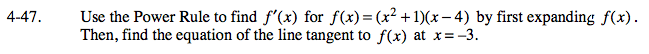### Home > CALC > Chapter 4 > Lesson 4.2.1 > Problem4-47

4-47.

Use the Power Rule to find f ′(x) for f(x) = (x2 + l )(x − 4) by first expanding f(x). Then, find the equation of the line tangent to f(x) at x = −3. Homework Help ✎Write the tangent line in point-slope form.

Which function, f(x) or f '(x), can be used to find the slope? Which can be used to find the point?